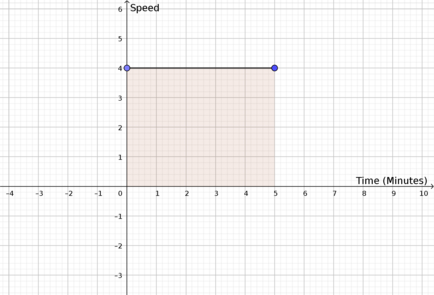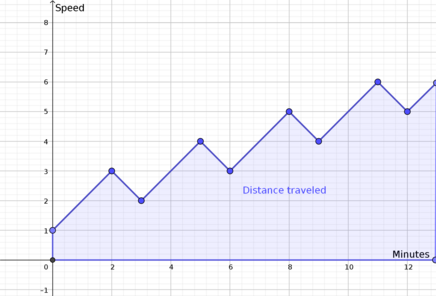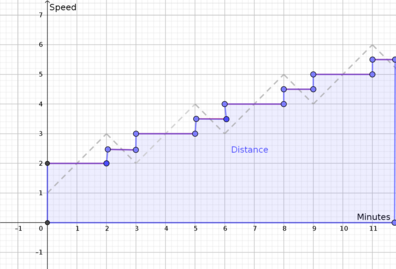# If a car goes at a speed of 1 mph, then accelerates to 3 mph in 2 minutes, then decelerates to 2 mph in 1 minute, then accelerates to 4 mph in 2 minutes, etc., then how many miles will it go when it reaches 60 mph?

• Problem:
If a car goes at a speed of $$1$$ mph, then accelerates to $$3$$ mph in $$2$$ minutes, then decelerates to $$2$$ mph in $$1$$ minute, then accelerates to $$4$$ mph in $$2$$ minutes, etc., then how many miles will it go when it reaches $$60$$ mph?

• @spaceblastxy1428 Interesting question! This is actually similar to a question that Prof. Loh covers in the Day 16 Your Turn question of Module 1! Can we assume that the acceleration is constant in this question?

I wasn't sure how long the car drove at $$1$$ mph for, so I just assumed that it immediately starts accelerating.

We can graph the speed in mph vs. time (in minutes) like this:The acceleration is constant at $$\pm 1 \text{ } \frac{\text{mph}}{\text{min}}$$ for each straight segment of the zigzag. From physics, we learn that

$$\text{distance} = \text{speed} \times \text{time}$$

(Though actually in physics, they use velocity instead of time, since velocity takes into account the direction of where an object is moving.)

Thus the distance can be interpreted as the area under a graph of speed versus time, like in the following example:Here the distance traveled is equal to

$$\text{ distance } = 4 \frac{\text{miles}}{\cancel{\text{hour}}} \times \frac{1}{12} \text{ } \cancel{\text{hour}} = \textcolor{red}{\frac{1}{3} \text{ miles }}$$

Even though our graph doesn't look as nice and simple as the one above, the distance traveled is also the same: it's the area under the speed versus time curve.The area above is equivalent to the area of the shape below, found by averaging the speed for each interval:Remember that we must convert the time duration of each interval from minutes to hour, to match the units of the speed (miles per hour). Thus we go at

\begin{aligned} 2 & \text{ mph for } \frac{1}{30} \text{ hour } \\ 2.5 & \text{ mph for } \frac{1}{60} \text{ hour } \\ 3 & \text{ mph for } \frac{1}{30} \text{ hour } \\ 3.5 & \text{ mph for } \frac{1}{60} \text{ hour } \\ 4 & \text{ mph for } \frac{1}{30} \text{ hour } \\ 4.5 & \text{ mph for } \frac{1}{60} \text{ hour } \\ 5 & \text{ mph for } \frac{1}{30} \text{ hour } \\ 5.5 & \text{ mph for } \frac{1}{60} \text{ hour } \\ 6 & \text{ mph for } \frac{1}{30} \text{ hour } \\ 6.5 & \text{ mph for } \frac{1}{60} \text{ hour } \\ \dots \\ 59 & \text{ mph for } \frac{1}{30} \text{ hour } \\ 59.5 & \text{ mph for } \frac{1}{60} \text{ hour } \\ \end{aligned}

In the question, it's not clear how long the car spends driving at $$60 \text{ mph},$$ so I'll just assume that once it reaches $$60 \text{ mph}$$ it no longer continues driving.

Thus the total distance, in miles, is

\begin{aligned} & 2 \times \frac{1}{30} + 2.5 \times \frac{1}{60} + 2 \times \frac{1}{30} + 2.5 \times \frac{1}{60} \\ & + 3 \times \frac{1}{30} + 3.5 \times \frac{1}{60} + 4 \times \frac{1}{30} + 4.5 \times \frac{1}{60} \\ \ldots \end{aligned}

I'll leave it to you to figure out how to simplify this expression!